currents of the individual branches of the circuit. The total resistance of a parallel circuit is equal to the reciprocal of the sum of the reciprocals of the individual resistances of the circuit."> Rules for parallel DC circuitsCustom SearchRules for series DC circuitsMatter, Energy, and Electricity NextRULES FOR PARALLEL DC CIRCUITS:

The same voltage exists across each branch of a parallel circuit and is equal to the source voltage.
The current through a branch of a parallel network is inversely proportional to the amount of resistance of the branch.
The total current of a parallel circuit is equal to the sum of the currents of the individual branches of the circuit.
The total resistance of a parallel circuit is equal to the reciprocal of the sum of the reciprocals of the individual resistances of the circuit.
The total power consumed in a parallel circuit is equal to the sum of the power consumptions of the individual resistances.

THE SOLUTION OF A COMBINATION CIRCUIT is
a matter of applying the laws and rules for series and parallel circuits as applicable.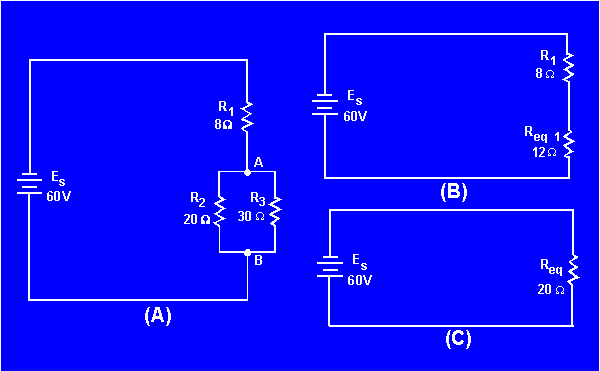ALL PARALLEL CIRCUITS ARE COMBINATION CIRCUITS when the internal resistance of the source is taken into account.

REDRAWING CIRCUITS FOR CLARITY is accomplished in the following steps:

Trace the current paths in the circuit.
Label the junctions in the circuit.
Recognize points which are at the same potential.
Visualize rearrangements, "stretching" or "shrinking," of connecting wires.
Redraw the circuit into simpler form (through stages if necessary).EQUIPMENT PROTECTION from short-circuit current is accomplished by use of fuses and other circuit protection devices.

A VOLTAGE DIVIDER is a series circuit in which desired portions of the source voltage may be tapped off for use in equipment. Both negative and positive voltage can be provided to the loads by the proper selection of the reference point (ground).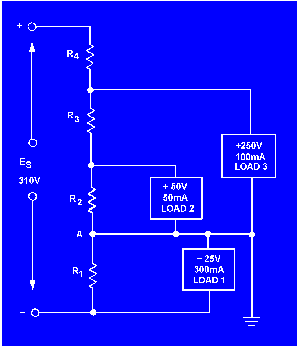ELECTRICAL SAFETY PRECAUTIONS must be observed. A fatal shock can occur from 0.1 ampere of current. Voltages as low as 30 volts have been recorded as causing sufficient current to be fatal.

ALL LIVE ELECTRICAL CIRCUITS shall be treated as potential hazards at all times.

ELECTRONIC OR ELECTRICAL EQUIPMENT discovered to be faulty or unsafe should be reported immediately to proper authority.

ELECTRICAL OR ELECTRONIC EQUIPMENT should be used and repaired by authorized personnel only.

A CO2 EXTINGUISHER should be used to extinguish electrical fires.

FIRST AID FOR ELECTRICAL SHOCK includes the following actions:

Remove the victim from the source of the shock.
Check the victim to see if the person is breathing.
If the victim is not breathing, give artificial ventilation. The preferred method is mouth-to-mouth.
CPR may be necessary if the heartbeat has stopped, but do not attempt this unless you have been trained in its use. OBTAIN MEDICAL ASSISTANCE AS SOON AS POSSIBLE.

ANSWERS TO QUESTIONS Q1. THROUGH Q61.

(a) DS1, the flashlight bulb(b) BAT, the dry cell

The path for current is incomplete; or, there is no path for current with S1 open.

A schematic diagram.

(a) Current increases(b) Current decreases.

(a) Current decreases(b) Current increases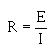1.25 amperes.

4 amperes.

Power.

By changing the circuit resistance or the voltage of the power source.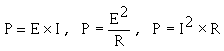6 amperes.

A wirewound resistor.

1 kilowatt.

8,952 watt hours or 8.952 kWh.

.942 (rounded to 3 places).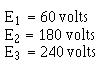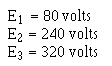The source voltage would have to be increased to 640 volts.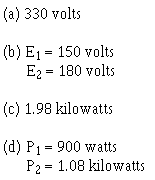The point at which current enters the resistor is assigned a negative polarity and the point at which current leaves the resistor is assigned a positive polarity.

2 amperes.

120 volts.

50 volts.

Zero volts.

A circuit where there is no longer a complete path for current flow.

An accidental path of low resistance which passes an abnormally high amount of current.

The internal (source) resistance of the battery will drop some of the voltage.

When the load resistance equals the source resistance.

50 percent.60 volts.

Total current in a series circuit flows through every circuit component but in a parallel circuit total current divides among the available paths.

Whether the current is entering the junction (+) or leaving the junction (-).Equivalent resistor or Req.

In both cases all the power used in the circuit must come from the source.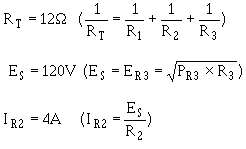PT = 60 W, ER2 = 10 V.

4Ω.

25Ω.

Because of the 2-volt drop across the internal resistance, only 48 volts
is available for the rest of the circuit.

(a) Total resistance increases, total current decreases(b) Total resistance becomes infinite, total current is equal to zero

(a) Total resistance decreases, total current increases(b) Total resistance decreases, total current increases

None.

The source voltage and load requirements (voltage and current).

45 mA Rule-of-thumb).

2 kΩ.

495 mA.

R1 is the bleeder resistor. Bleeder current must be known before any of the remaining divider resistor ohmic values can be computed.

(a) By adding the bleeder current (IR1) and the current through load 1(b) By subtracting the voltage of load 1 from the voltage of load 2

1.35 watts.

The series-parallel network drops the remaining source voltage and is used to take the place of a single resistor (75 ohms) when the required ohmic value is not available in a single resistor.

R3 = 2 watts; R5 = 6 watts.

The ground (reference point) is placed in the proper point in the voltage divider so that positive and negative voltages are supplied.

NEVER! All energized electric circuits should be considered potentially dangerous.

You should immediately report this condition to a qualified technician.

Only trained, qualified personnel.

Privacy Statement - Copyright Information. - Contact UsIntegrated Publishing, Inc.